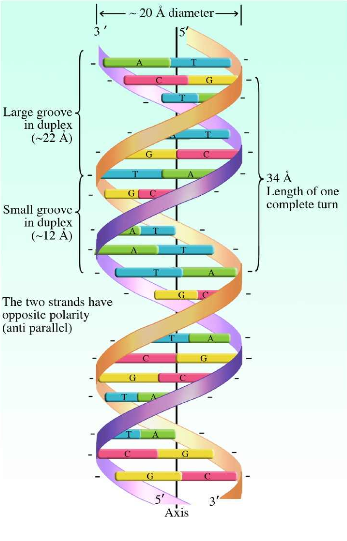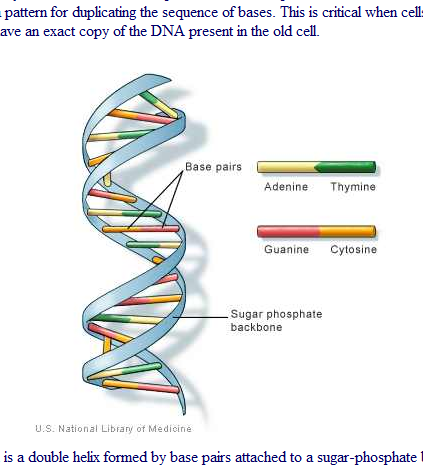Welcome Guest!
Sunday, 03.26.2023, 4:08 PM

Total online: 1
Guests: 1
Users: 0

## Calendar

 «  March 2023  » Su Mo Tu We Th Fr Sa 1 2 3 4 5 6 7 8 9 10 11 12 13 14 15 16 17 18 19 20 21 22 23 24 25 26 27 28 29 30 31

## Site friends

RD-blog-number-2463

Do molecules do math calculations?
Why not?
They do everything else necessary for human biological existence.

Let's look at Nature's CLUES that may LINK DNA  to the algebra quadratic equation ...and bio-math symbolic expressions of thought.

### DNA - Wikipedia, the free encyclopedia

en.wikipedia.org/wiki/DNA
Deoxyribonucleic acid (DNA) molecules are informational molecules encoding the genetic instructions used in the development and functioning of all known ...We see the message about the axis ...and are reminded of high school algebra quadratic equation and the graph of a parabola on a piece of paper with an x, y axis.The double-helix implies number 2.

In mathematics, a quadratic equation is a univariate polynomial equation of the second degree. A general quadratic equation can be written in the form$ax^2+bx+c=0,\,$

where x represents a variable or an unknown, and a, b, and c are constants with a ≠ 0.f (x) = x2x − 2 = (x + 1)(x − 2) of a real variable x, the x-coordinates of the points where the graph intersects the x-axis, x = −1 and x = 2, are the solutions of the quadratic equation: x2x − 2 = 0.

A quadratic equation with real or complex coefficients has two solutions, called roots. These two solutions may or may not be distinct, and they may or may not be real.

Having$ax^2+bx+c=0,\,$

the roots are given by the quadratic formula$x=\frac{-b \pm \sqrt {b^2-4ac}}{2a},$

where the symbol "±" indicates that both$x=\frac{-b + \sqrt {b^2-4ac}}{2a}\quad\text{and}\quad x=\frac{-b - \sqrt {b^2-4ac}}{2a}$

are solutions of the quadratic equation.

The key symbols above are
4ac --------->  reminders of 4 DNA nucleotides of  Deoxyribonucleic acid

4ac.....subset symbols.........4.......................................................ac

thus suggesting that the  DNA description be enhanced to include BIO-MATH attributes.

en.wikipedia.org/wiki/DNA
Deoxyribonucleic acid (DNA) molecules are  informational molecules encoding the genetic instructions used in the development and functioning

functioning --> function + ing --> math function integers ...bio-math abilities

What are some more bio-math possibilities.

Brain axons --word axon --> ax + o + n  --> suggesting the brain molecules have numeric abilities

ax --> the main math equation
0 --> the equation = 0
n --> may ne an exponent or a subscript

Case 1 --> If n is an exponent ..such as 2 ...the axon --> suggests the equation$ax^2+bx+c=0,\,$

Case 2 --> If n is a subscript  ....then that suggest a set of linear equations ...like a square matrix of order n.

The existence of the double-helix ...a  2 structure .... makes the quadratic equation attractive.

### What are the 4 nucleotides for DNA

What are the 4 nucleotides for DNA? In: Genetics [Edit categories]. Answer:

The 4 nucleotides in DNA are:

G =  Guanine
C = Cytosine
T  = Thymine ...$x=\frac{-b \pm \sqrt {b^2-4ac}}{2a},$

We see the algebra answer component   4ac --> 4 DNA nucleotides with a = adenine and c =cytosine

adenine X exponent 2    +  b X  + cytosine  =  o

What is b ?
Could it symbolize base pair ..symbolic life of DNA?
Could it symbolize some bridge between the 2 strands?
Could it be the binary paired strands?$x=\frac{-b \pm \sqrt {b^2-4ac}}{2a},$

The plus/minus symbol could be equivalent to the left side strand and the right side strand of the binary pair ---> DOUBLE-Helix.
--> The two strands have opposite polarity  ...... that equivalent  to the plus/minus sign in the equation.Additional CLUES involve the word identifier for  DNA ....and numbers 16 and 4.

alphabet data symbol  ----> D e o x y r i b o n  u  c  l  e  i  c  ...... A c i d
DNA computer address ---> 1....3 ...5...7...9....11...13.......16 ---> 1.....4

Above we see the Base 16 hexadecimal nature of the living  word--> Deoxyribonucleic
In mathematics and computer sciencehexadecimal (also base 16, or hex) is a positional numeral system with a radix, or base, of 16. It uses sixteen distinct symbols,$ax^2+bx+c=0,\,$

we see the algebra answer component   4ac --> 4 DNA nucleotides with a = adenine and c =cytosine

Could the X varable in the equation ....be an abbreviation for BASE 16 HEX cosidering the 16 alphabet letters of word:  Deoxyribonucleic

adenine  HEX exponent 2    +  b  HEX  + cytosine  =  o

What is b ?
Could it symbolize base pair ..symbolic life of DNA?
Could it symbolize some bridge between the 2 strands?
Could it be the binary paired strands?$x=\frac{-b \pm \sqrt {b^2-4ac}}{2a},$

Thus we have a few starting pieces to an interesting puzzle.

Additional alpha/numeric pieces of the biochemistry WORDS.... their alphabet and number components

Adenine --subset symbols nine = 9
Guanine --> subset number nine = 9

Somehow this gets involved in some bio-math calculation.

At the moment we see  9 x  9 = 81.
Let's look at IBM system 370  and the IBM  8100 DPCX.

Let's convert the copper-wire computer into a
human bio-computer ..... in other words...let's take the copper-wire systems and their SYMBOLIC architecture  .....
and by reading those IBM manuals into our eyes/optical nerve --> route information to our INTERNAL  bio-chemistry department with NATURE's thought team ....

we see the
IBM  8100 DPCX
graphic shape of number 8 looks like letter B
graphic shape of number 5 look like S --> System equivalence

Thus 8100D PCX  ---> the ade.nine and Gua.nine role in  81 --> Blood math
........B100D Person Computer bio-alpha/numeric math model X

Thus we see that biochemistry has evolved into 2 major areas: the biology processes and the symbolic life parallel processses.
This was announced by the Darwin selection of  newspaper communication theorists .... Patty Hearst and her Darwinian experiences  with the Symbolic ARMY ..Symboise Army and
and the DNA social science EVENT ....ki.DNA.pping  --> DNA parallel processing ......signal from the 1970's message processing EVENT.

Let's change our VIEW...

Let's assume the universe was a mathematical symbolic  structure with atoms of the periodic atomic table ....but before these  esoteric DNA molecules existed.
Nature's molecular engineering teams ...millions of years ago ...were just like modern engineers.
Modern engineers do their math calculations,  various simulation studies, and make flowcharts and drawings of their proposed structures. etc ......

What if...Nature's  design engineers started with the quadratic equation model...design   ...... and then constructed  the biochemistry DNA  molecule in accordance with the algebra-geometry engineering model.
Of course, Nature added other things ... such as the  biochemistry .... chemistry processes.

Thus the quadratic equation ...as a axiomatic origin ...... gives the logical deduction -->of many DNA numeric features and names.